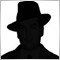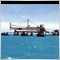• 概述
• 评论 (4)
• 评论 (8)

Adjustable Fractal is a modification of Bill Williams' Fractals indicator.

The fractal consists of a two sets of arrows - up (upper fractals) and down (lower fractals). Each fractal satisfies the following conditions:

• Upper fractal - maximum (high) of a signal bar exceeds or is equal to maximums of all bars from the range to the left and to the right;
• Lower fractal - minimum (low) of a signal bar is less or equal to minimums of all bars from the range to the left and to the right.

Unlike a standard indicator, Adjustable Fractal allows setting the number of bars to find fractals to the left and to the right of desired bars. This allows to flexibly determine the presence of fractals, for example during a long upward or downward movement.

### Inputs

• Bars before - number of bars before a fractal used in calculation;
• Bars after - number of bars after a fractal used in calculation.

You can set the arrows' color and size separately for upper and lower fractals on Colors tab.17225
Nikolay Gaylis 2019.01.14 06:1112010
2017.12.10 17:295438
2017.11.09 03:026084
2016.12.21 06:34